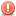CodeGuru Home VC++ / MFC / C++ .NET / C# Visual Basic VB Forums Developer.com

# Thread: I need help with a date class.

1.Junior MemberJoin Date
Jan 2009
Posts
24

##I need help with a date class.

I need help with this code:

Code:
```#ifndef DATE_H
#define DATE_H

#include <iostream>
#include <string>

using namespace std;

class date
{
private:
enum m_e_month                    //member enumeration named month
{
January=1,
Febuary=2,
March=3,
April=4,
May=5,
June=6,
July=7,
August=8,
September=9,
October=10,
November=11,
December=12
};
m_e_month m_m_e_month_month;                    //its a member variable m_ of type (m_e_month) named month
unsigned short int m_sh_I_year;
unsigned short int m_sh_I_day;
void makeyear()
{
cout<<"enter the year:";
int a;
cin>>a;

}
void makemonth()
{
bool redo=1;
cout<<"Enter the month's name it must be capitalied.";
while(redo)
{
string tmonth="0";
cin>>tmonth;                    //It set to 0 so is assumes nothings going to happen. If it weren't here thaen and infinite loop would start.
redo=0;                   //I have to use if and elses because switchs don't work with emums.
if(tmonth=="January")
{m_m_e_month_month=January;}
else if(tmonth=="Febuary")
{m_m_e_month_month=Febuary;}
else if(tmonth=="March")
{m_m_e_month_month=March;}
else if(tmonth=="April")
{m_m_e_month_month=April;}
else if(tmonth=="May")
{m_m_e_month_month=May;}
else if(tmonth=="June")
{m_m_e_month_month=June;}
else if(tmonth=="July")
{m_m_e_month_month=July;}
else if(tmonth=="August")
{m_m_e_month_month=August;}
else if(tmonth=="September")
{m_m_e_month_month=September;}
else if(tmonth=="October")
{m_m_e_month_month=October;}
else if(tmonth=="November")
{m_m_e_month_month=November;}
else if(tmonth=="December")
{m_m_e_month_month=December;}
else
}
}
void makeday()
{
int numofdays;
int placeholder;
placeholder=m_m_e_month_month;                    //again I can't use a switch statement due to repetitivness and it is not compatible
if ((placeholder==1)||(placeholder==3)||(placeholder==5)||(placeholder==7)||(placeholder==8)||(placeholder==10)||(placeholder==12))
{
numofdays=31;
}
else if ((placeholder==2))                    //this is just for febuary.
{
numofdays=28;
if(m_sh_I_year&#37;4==0)                    //febuary has leap years
{
numofdays=29;
}
}
else
{
numofdays=30;                   //this is for all the other months
}
m_sh_I_day=0;                    //this is so the so while loop doesn't show the invalad thingy.
do
{
if (m_sh_I_day>numofdays)
{
}
cout<<"enter the day(number)";
cin>>m_sh_I_day;
}while(m_sh_I_day>numofdays);
}
public:
date(int a=0, m_e_month b=January, int c=-1)
{
m_sh_I_year=a;
m_m_e_month_month=b;
m_sh_I_day=c;
}
friend &ostream operator<<(ostream &out, date &adate);
friend &istream operator>>(istream &in, date &adate);
};

{
return out;
}

{
}

#endif```
when I try to compile it I get these bugs

ISO C++ forbids declaration of `ostream' with no type
`ostream' is neither function nor member function; cannot be declared friend
expected `;' before "operator"
ISO C++ forbids declaration of `istream' with no type
`istream' is neither function nor member function; cannot be declared friend
expected `;' before "operator"
expected constructor, destructor, or type conversion before "operator"
expected constructor, destructor, or type conversion before "operator"
Last edited by E-man96; February 13th, 2009 at 02:52 AM.Reply With Quote

class, date, iostream, istream, ostream####Posting Permissions

• You may not post new threads
• You may not post replies
• You may not post attachments
• You may not edit your posts
•